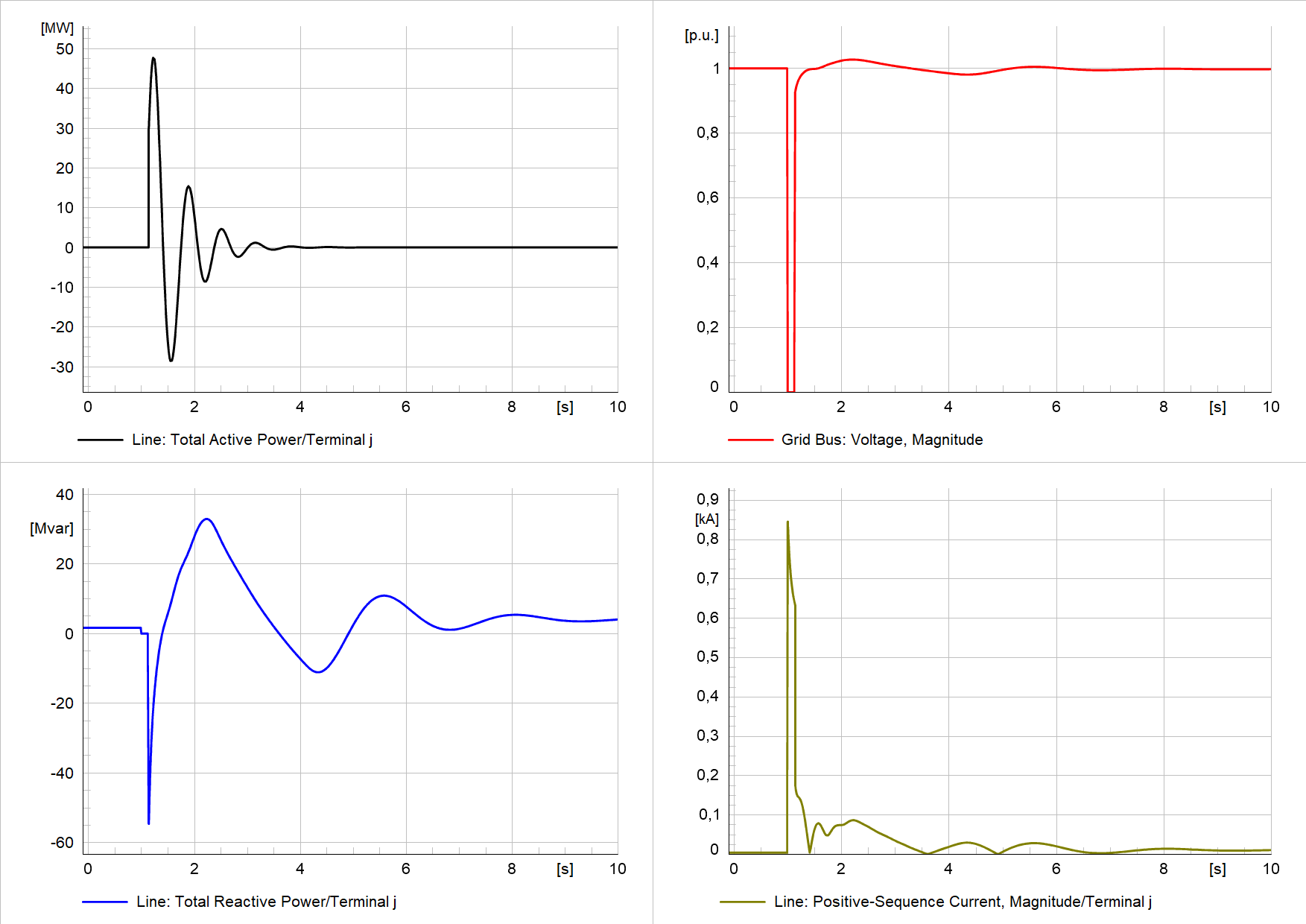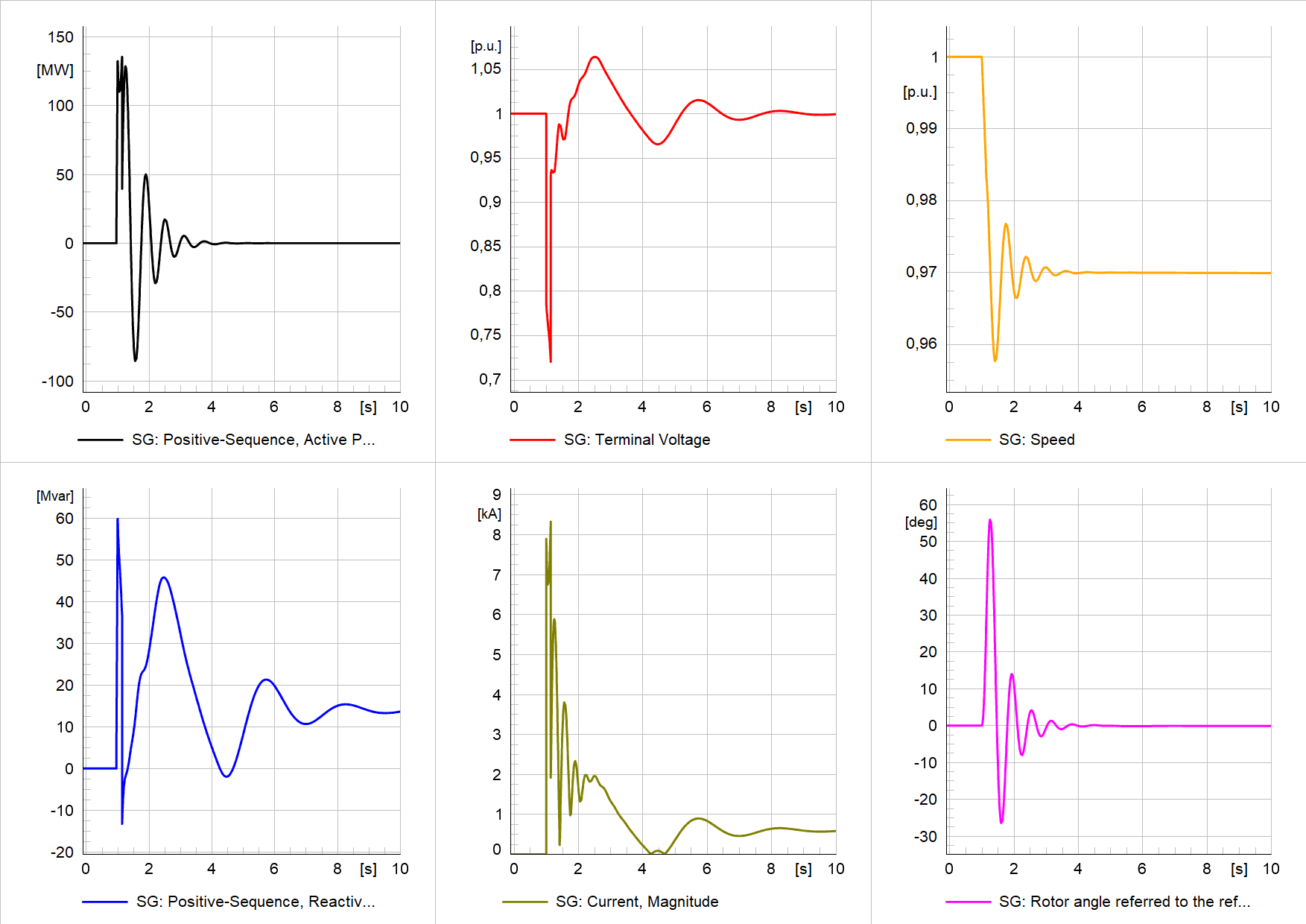# A template for fault-ride-through (FRT) analysis of synchronous generators/condensers

The importance of grid connection studies increases around the world. TSOs focus more on the grid impact of new generation units as the energy transition moves forward. Adding more converter-based units changes the power system dynamics fundamentally. That’s also why most TSOs require a fault-ride-through (FRT) analysis of prospective units before approval.

Similarly important is the rate-of-change-of-frequency (RoCoF) analysis. Check this post for more information: A template for rate-of-change-of-frequency (RoCoF) analysis of synchronous generators/condensers

Here, I provide a template for fault-ride-through analysis of synchronous generators including a step-by-step guide on how to use it. It is also suitable for synchronous condensers because they are like synchronous generators, just without a prime mover. The template is implemented in DIgSILENT PowerFactory.

## Single-machine infinite-bus (SMIB) concept

The PowerFactory model includes a single-machine infinite-bus model. The model consists of a synchronous generator, transformer, line/cable, and the external grid as shown below.

## Synchronous generator excitation and voltage control

To model the excitation and voltage control of the synchronous generator, I used the IEEE ST3C model. This is a purely arbitrary choice as the model needs to be adapted according to the real excitation and voltage control system of the investigated generator.

## Study cases for the fault-ride-through analysis

Generally, grid connection studies assess the impact of the unit at the Point of Connection (PoC). The following study cases present a selection of scenarios that are usually required.

### Study case 1 – three-phase short circuit (fault impedance = 0)

Three-phase short-circuit at the PoC without fault impedance. This usually marks the worst-case scenario.

The most important results are the generator variables and the variables at the PoC. The plot below shows the results for the generator variables: active/reactive power, terminal voltage, generator current, speed, and rotor angle.Simulation results of study case 1: Generator variables –active/reactive power, terminal voltage, generator current, speed, and rotor angle

In the plot below you can find the results for the PoC. Here it is important that the branch variables active/reactive power and current represent the contribution from the generator (through the connected line). The voltage is a bus variable and given for the PoC (which I call Grid Bus here).Simulation results of study case 1 at PoC –active/reactive power and current (contribution from the unit), and voltage at PoC

### Study case 2 – three-phase short circuit (fault impedance ≠ 0)

Three-phase short-circuit at the PoC with fault impedance.

Sometimes it is required to simulate a short-circuit with a specific retained voltage at the PoC. In this case, the fault impedance needs to be set so that the required retained voltage is achieved. To do that, I have programmed a short Python script where you can choose the retained voltage and it will find the corresponding fault impedance.

In the following, you can see the results for a three-phase fault with a retained voltage of 0.7 pu at 1.1 seconds (100 ms after fault inception) at the PoC. The fault impedance was calculated as R = 49.50 Ω and X = 4.95 Ω (R/X = 10). The fault impedance ratio can be specified by the user (please refer to the code snippet below the two plots).

The plot below shows the generator variables: active/reactive power, terminal voltage, generator current, speed, and rotor angle.Simulation results of study case 2: Generator variables –active/reactive power, terminal voltage, generator current, speed, and rotor angle

In the plot below you can find the results for the PoC. Here it is important that the branch variables active/reactive power and current represent the contribution from the generator (through the connected line). The voltage is a bus variable and given for the PoC (which I call Grid Bus here).

Looking at the voltage (top right) we can see that the retained voltage at 1.1 seconds is equal to 0.7 pu as desired. The Python script is really handy to get the right fault impedance for a given retained voltage, especially if you consider an R/X (or X/R) ratio.Simulation results of study case 2 at PoC –active/reactive power and current (contribution from the unit), and voltage at PoC

#### Code snippet of the Python script to calculate the fault impedance

Below you can find a code snippet (full code included in the download) that shows the inputs of the Python script to calculate the corresponding fault impedance for a specified retained voltage. You can specify the retained voltage (u_target) and at which time it should reach the value (t_target). It is even possible to specify different modes in terms of impedance ratios, e.g. R/X or pure X.

``````#%% inputs for the impedance calculation

#project and study case
projName='_TSI_FRT_template'
study_case = '02-3p-fault-PoC_impedance (ret. voltage)'

#target retained voltage and time
u_target = 0.7 # (pu) retained voltage target
t_target = 1.1 # (s) target time for retained voltage

mode = 1 # 1=R/X, 2=X/R, 3=R(X=0), 4=X(R=0)
ratio = 10 # ratio of fault impedance (if 1 or 2 chosen above)``````

## A step-by-step guide to carry out fault-ride-through analysis

In this section, I provide a step-by-step guide on how to do a fault-ride-through analysis on your own.

2. Change the parameters of the equipment
• Generator
• Transformer
• Line
• External Grid
3. If necessary, change the voltages of the buses (SG Bus, HV Bus, Grid Bus).
4. Change the common model of the excitation and AVR and adapt the parameters to comply with your equipment
5. Set the active and reactive power of the synchronous generator as desired
6. Set the voltage setpoint at the synchronous generator terminal through the station control (the external grid controls the initial voltage at the synchronous generator terminal)
7. If necessary, change the short-circuit events, i.e. fault inception and clearing time.
8. Run the simulation for study case 1 (fault impedance = 0): Initial conditions + RMS simulation
9. Open the Python script and define:
• Path to your PowerFactory installation (line 32 in the script)
• project name
• study case
• target retained voltage
• target time for the retained voltage
10. Run the Python script (don’t forget to close PowerFactory, otherwise you can’t run the script)
11. Open PowerFactory (restart Python console before opening PowerFactory to avoid license problems) and run the simulation for study case 2

I hope you enjoyed this article. If so, you might also like the following: Automatized short-circuit analysis with Python in DIgSILENT PowerFactory

More insights to follow in the next article.

Michael